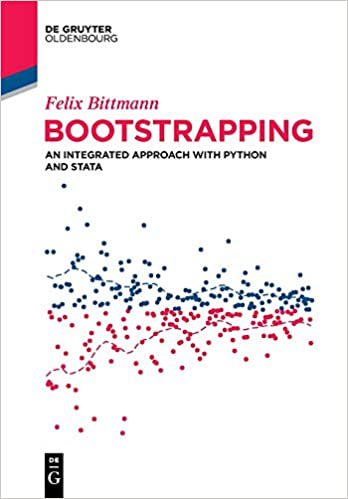# Bootstrapping: An Integrated Approach with Python and Stata

Bootstrapping: An Integrated Approach with Python and Stata, by Felix Bittmann, is a great resource for students and researchers who want to learn and apply bootstrap methods.

The text begins with a clear introduction to foundational statistics on which bootstrapping methods rely. Bittmann then walks users through the logic behind bootstrapping as well as the process. The book includes discussion of confidence intervals and hypothisis testing as well as a chapter focused on bootstrap considerations related to regression models.

The final chapter demonstrates how researchers can perform bootstrapping using Stata and Python. Examples range from straightforward use of Stata’s bootstrap prefix and vce(bootstrap) option to more advanced techniques such as writing a program for resampling residuals. With this knowledge, readers will be ready to apply bootstrapping in their own analyses using Stata.

BASIC STATISTICAL CONCEPTS

Arithmetic mean
Standard deviation
Standard error
Confidence intervals
Applied example
P-values
Testing for normality
Probability density functions
Bias and variance
Normality and confidence intervals revisited

BOOTSTRAPPING

Working example
Requirements
Diagnostics
Unique values
Distribution of bootstrap-results
Rate of convergence
The logic of bootstrapping
Failures of the bootstrap

CONFIDENCE INTERVALS

Normal intervals
Percentile intervals
Bias corrected intervals (BC)
Bias corrected and accelerated intervals (BCa)
Double bootstrap
Transformations
Summary and simulation

HYPOTHESIS TESTING

The logic of hypothesis tests
Confidence intervals
Sampling in accordance with the null
Permutation tests
Paired permutation tests
Boot or permute?
Power calculations

REGRESSION MODELS

Sampling observations
Sampling residuals
Dealing with outliers
Predicting outcomes and bagging

APPLIED PROGRAMMING EXAMPLES

Python
Basics
All confidence intervals
Permutation tests
Importing data
Appendix
Stata
Descriptive statistics
Estimating models
Writing own programs
Permutation tests
Longitudinal and cluster analyses
Computational speed